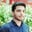Trusted answers to developer questions
Trusted Answers to Developer Questions

Related Tags

pandas

# What is the DataFrame.radd() method in pandas?Salman Yousaf

Grokking Modern System Design Interview for Engineers & Managers

Ace your System Design Interview and take your career to the next level. Learn to handle the design of applications like Netflix, Quora, Facebook, Uber, and many more in a 45-min interview. Learn the RESHADED framework for architecting web-scale applications by determining requirements, constraints, and assumptions before diving into a step-by-step design process.### Overview

The DataFrame.radd() method is used to perform addition between other objects and this DataFrame. The other argument can be a DataFrame, Series, Constant, or a scalar.

This method is similar to DataFrame.add(). However, the DataFrame.radd() method is essentially added to perform addition between odd objects Objects of different classes or modulesand DataFrame.

### Syntax

DataFrame.radd(other, axis='columns', level=None, fill_value=None)

### Parameters

• other: It can either be a DataFrame, series, sequence, or a constant.
• axis='columns': This specifies whether we have to compare values as index or column. For series, values are matched by the series index. It can either be 'index' or 'column'. It is 'column' by default.
• level=None: It helps to broadcast dimensions when multi-indexing is required for multiple levels.
• fill_value=None: It is used to fill existing empty values with NaN.

### Return value

This method returns a DataFrame after performing arithmetic addition between the this DataFrame and the other argument value.

Let's look at some examples of the radd() method using different argument values.

### When other is a DataFrame:

# When Both this & Argument Are DataFrames# import pandas moduleimport pandas as pd# DataFrame containing three tests marks for# mathematics and computerother = pd.DataFrame({'Mathematic': [90, 33, 49],                   'Computer': [78, 95, 85]},                  index=['Test#1', 'Test#2', 'Test#3'])# Another DataFrame with different marksdf = pd.DataFrame({'Mathematic': [15, 45, 71],                   'Computer': [66, 79, 85]},                  index=['Test#1', 'Test#2', 'Test#3'])# radd() will return a resultant DataFrame# print addition on consoleprint(df.radd(other))

### Explanation

• Lines 6–8: We create a DataFrame containing three records of exam marks for mathematics and computer subjects.
• Lines 10–12: We create another DataFrame having a record of exam marks for two subjects i.e. Mathematics and Computer
• Line 15: We use the df.radd(other) method to evaluate addition between other as well as df DataFrames.

### When other is a series

# When one is series and other is Dataframe# import pandas moduleimport pandas as pd#Created a marks DataFramedf = pd.DataFrame({'Microsoft': [19000, 33000, 4900],                   'Google': [88000, 78000, 85000]},                  index=['Data Scientist($)', 'Software Engineer($)', 'Product Manager($)'])# created a seriesbonus = pd.Series([100, 200, 300], index=['Data Scientist($)', 'Software Engineer($)', 'Product Manager($)'])# evaluate addition between DataFrame and seriesprint(df.radd(bonus, axis='index'))

### Explanation

• Lines 5–7: We create a DataFrame df that contains salaries of different ranks in Microsoft and Google.
• Line 9: We create a series of three values as a bonus income.
• Line 11: We invoke the radd() method to add data frame df and series bonus values by index.

# When one is series and other is Dataframe# import pandas moduleimport pandas as pd#Created a marks DataFramedf = pd.DataFrame({'Microsoft': [19000, 33000, 4900],                   'Google': [88000, 78000, 85000]},                  index=['Data Scientist($)', 'Software Engineer($)', 'Product Manager($)'])# evaluate addition between DataFrame and constantprint(df.radd(200)) ### Explanation • Lines 5–7: We create a DataFrame df that contains salaries of different ranks in Microsoft and Google. • Line 9: We invoke df.radd() with constant 200 as the other argument. It will add 200 at each index and return an updated DataFrame. Note: DataFrame.radd() is different from DataFrame.add() because it is designed to perform addition between Pandas DataFrame and Others (DataFrame, Scalar, constant, or series). It is equivalent to other + dataframe arithmatic operator. # When one is series and other is Dataframe# import pandas moduleimport pandas as pd#Created a marks DataFramedf = pd.DataFrame({'Microsoft': [19000, 33000, 4900], 'Google': [88000, 78000, 85000]}, index=['Data Scientist($)', 'Software Engineer($)', 'Product Manager($)'])# addition with arithmatic operatorprint(df + 200)
• Lines 5–7: We create a DataFrame df that contains salaries of different ranks in Microsoft and Google.
• Line 9: We use df + 200 to increment each index of DataFrame with 200 and return an updated DataFrame.

RELATED TAGS

pandas

CONTRIBUTORSalman Yousaf

Grokking Modern System Design Interview for Engineers & Managers

Ace your System Design Interview and take your career to the next level. Learn to handle the design of applications like Netflix, Quora, Facebook, Uber, and many more in a 45-min interview. Learn the RESHADED framework for architecting web-scale applications by determining requirements, constraints, and assumptions before diving into a step-by-step design process.Keep Exploring

Learn in-demand tech skills in half the time